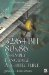Chapter 11: BranchlessOverview

Okay, we learned how to branch in the last chapter. We also learned about some branchless coding methods such as the butterfly switch and value setting using bit blending. Now let's learn how to make decisions without branching. You have already learned instructions such as the bit test and set. Here you will be shown how to use masking logic to get the same result as one would with the use of branching. The first item to learn is that the MSB (negative bit #31) and Carry bits are your friends ! Two values can be subtracted to obtain a positive or negative threshold, and then the resulting state of the MSB can be arithmetically shifted to create a mask of all ones or zeros. That mask can be used to mask or blend bits.There is only one trick, however. The values need to be one bit less than the data size so as to take advantage of the MSB. Data could be shifted by the bit width of the data, but on some older embedded processors there are penalties for each shifted bit.

#define UINT_MAX_BITS   (sizeof(uint)   <<3)         // 32-bit value = 32 #define INT_MAX_BITS    ((sizeof(int)   <<3)1)      // 32-bit value = 31 #define UCHAR_MAX_BITS  (sizeof(uchar)  <<3)         // 8-bit value = 8 #define CHAR_MAX_BITS   ((sizeof(char)  <<3)1)      // 8-bit value = 732/64-Bit 80x86 Assembly Language Architecture
ISBN: 1598220020
EAN: 2147483647
Year: 2003
Pages: 191

Similar book on Amazon